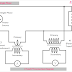Hey, in this article we are going to see CT and PT connection diagrams. CT stands for Current Transformer and the PT stands for potential transformer. As the name suggests the current transformer or CT operates with electrical current whereas the potential transformer or PT operates with electrical voltage or potential. Both of them are known as instrument transformers and are mainly used for measurement purposes such as electrical current flow and system voltage.

The potential transformer is almost the same as a power transformer or distribution transformer just the difference is it is designed for a low rating. Generally, the primary winding of a potential transformer is designed 100 to 500 Volt-ampere and the secondary of the potential transformer is designed 115 to 120 volt-ampere. The primary winding of the PT has more number of turns than the secondary winding or basically, it is a step-down transformer. When it is connected to a high voltage system it steps down the voltage and by calculating the turns ratio we can measure the actual system voltage.

On the other hand, the current transformer is basically a step-up transformer. It has very few turns in its primary winding and a large number of turns in the secondary winding. There are two types of current transformer one is to be directly connected to the circuit. Generally, the primary winding of the current transformer is to be connected in series with the line whose current is to be measured.

The other type of CT is the sensing type, it does not need to connect directly to the power lines. Basically, the conductive line whose current is to be measure placed or should go through the core of the CT, and the CT will use the line as the primary winding.

## CT and PT Connection Diagram For Single Phase System

Here, you can see the connection of CT(Current Transformer) and PT(Potential Transformer) for a single-phase system.

Here, you can see the primary winding of the CT is connected in series with the line and the secondary winding of the CT is connected across the ammeter. On the other hand, the primary winding of the PT is connected in parallel with the line. And the secondary of the PT is connected across a voltmeter. Now let's see the connection diagram for sensing type CT that does not have any direct connection with the power line. Here, basically core balance current transformer(CBCT) is used. In case of a PT, there is no sensing type, it always be physically connected to the system.

## CT and PT Connection Diagram For Three Phase System

Here, you can see the connection diagram of CT and PT for a Three-Phase system where three live wires and a neutral wire are available.

Here, you can see the one terminal of each CT is connected together and connected to the ground. One terminal of each ammeter is also connected here. This is called star connection. The rest terminal of the CT is connected to the ammeter one terminal respectively. On the other hand, you can see, one terminal of each PT is connected together and connected to the neutral and ground. Same as one terminal of the secondary winding of each PT is connected together. The Potential Transformers are also connected in Star Configuration. Here, polarity is not so much important thing but the connection of a particular meter should be accurate. That means different connection should be done to measure phase voltage and line voltage.

CT and PT Connection Diagram ExplainedReviewed by Author on April 05, 2022 Rating: 5